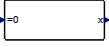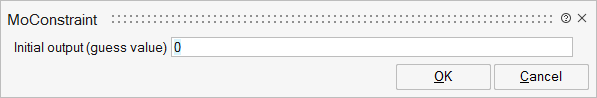# MoConstraint

This block outputs a Modelica signal so that its input remains zero.CustomBlocks

## Description

This block outputs values so that its input remains zero. This constraint works only if there is a path in the diagram from the output to the input of the block. If F is the function that corresponds to this path, the block solves the equation F(x)=0 where x denotes the output of the block. If the solver cannot find an initial solution, you can provide an initial guess value for the output. If F(x)=0 has several solutions, the initial guess helps the solver to select the desired solution.

## ParametersNameLabelDescriptionData TypeValid Values

y0

Initial output (guess value)

Scalar

## Ports

NameTypeDescriptionIO TypeNumber

x

implicit

output

1

=0

implicit

input

1